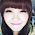## Saturday, June 11, 2011

### Real number Test paper

Real number Test paper
1. Write whether every positive integer can be of the form 4q + 2, where q is an

2. “The product of two consecutive positive integers is divisible by 2”. Is this statement
true or false? Give reasons.

3. “The product of three consecutive positive integers is divisible by 6”. Is this statement

4. Write whether the square of any positive integer can be of the form 3m + 2, where
m is a natural number. Justify your answer.

5. A positive integer is of the form 3q + 1, q being a natural number. Can you write its
square in any form other than 3m + 1, i.e., 3m or 3m + 2 for some integer m? Justify

6. The numbers 525 and 3000 are both divisible only by 3, 5, 15, 25 and 75. What is

7. Explain why 3 × 5 × 7 + 7 is a composite number.

8. Can two numbers have 18 as their HCF and 380 as their LCM? Give reasons.

9. Without actually performing the long division, find if 987/10500
will have terminating or non-terminating (repeating) decimal expansion. Give reasons for your answer.

10. A rational number in its decimal expansion is 327.7081. What can you say about
the prime factors of q, when this number is expressed in the form p/q ? Give reasons.

1.Character is like a tree and reputation like a shadow. The shadow is what we think of it; the tree is the real thing. See the link below for more info.

#real
www.mocsbar.com

2.I enjoy reading your articles. You really have a wonderful blogs. Keep up the good work. Thank you also for the information!

Cara
www.gofastek.com

3.Love it! Very interesting topics, I hope the incoming comments and suggestion are equally positive. Thank you for sharing this information that is actually helpful.

ufgop.org
ufgop.org